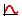# Fit a Curve by Estimating UTS

An empirical formula can be used to estimate SN/EN data from ultimate tensile strength (UTS) and Young's modulus (E).

1. From the Assign Material dialog, click the My Material tab and select your created material.
2. Select Estimate from UTS as the input method.
3. Clickto view the model description.
4. Enter a value for UTS and Elastic modulus.
5. Click Estimate.

## SN Properties

$SRI1$
Fatigue strength coefficient. It is the stress amplitude intercept of the SN curve at 1 cycle on a log-log scale.
$b1$
The first fatigue strength exponent. The slope of the first segment of the SN curve in log-log scale.
$Nc1$
In one-segment SN curves, this is the cycle limit of endurance (See Nc1 in Figure 2). In two-segment SN curves, this is the transition point (see Nc1 in Figure 4).
$b2$
The second fatigue strength exponent.

## EN Properties

$Sf/{{\sigma }^{\prime }}_{f}$
Fatigue strength coefficient.
$b$
Fatigue strength exponent.
$c$
Fatigue ductility exponent.
$Ef/{{\epsilon }^{\prime }}_{f}$
Fatigue ductility coefficient.
$Np/{n}^{\prime }$
Cyclic strain-hardening exponent.
$Kp/{K}^{\prime }$
Cyclic strength coefficient.
${N}_{c}$
Reversal limit of endurance. One cycle contains two reversals.
$S{E}_{e}$
Standard Error of Log(N) from elastic strain.
$S{E}_{p}$
Standard Error of Log(N) from plastic strain.